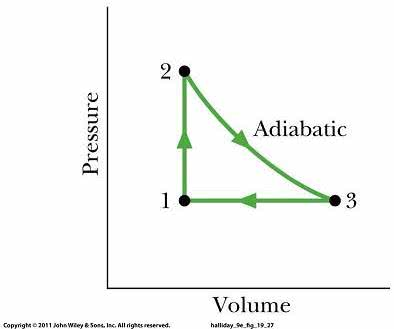1
0
watching
188
views

#Figure below shows a cycle undergone by 1.00 mol of an idealmonatomic gas. The temperaturesare T1 = 300 K, T2 = 600 K, and T3 = 455 K.For 1 ? 2, what are(a) (2 points) heat Q?(b) (2 points) The change in internal energy ?Eint?(c) (2 points) The work done W?For 2 ? 3, what are(d) (1 points) Q?(e) (2 points) ?Eint?(f) (2 points) W?For 3 ? 1, what are(g) (2 points) Q?(h) (2 points) ?Eint?(i) (2 points) W?For the full cycle, what are(j) (2 points) Q?(k) (2 points) ?Eint?(l) (2 points) W?The initial pressure at point 1 is 1.00 atm (= 1.013 Ã 105 Pa).What are the(m) (2 points) volume?(n) (2 points) Pressure at point 2?(o) (2 points) Volume?(p) (1 points) Pressure at point 3?